# Number of Waveform Samples VI

## LabVIEW 2018 Help

Edition Date: March 2018
Part Number: 371361R-01
View Product InfoLabVIEW 2015 HelpLabVIEW 2016 HelpLabVIEW 2017 HelpLabVIEW 2018 HelpLabVIEW 2019 Help

Owning Palette: Analog Waveform VIs and Functions

Requires: Base Development System

Returns the number of data elements in the waveform. You must manually select the polymorphic instance you want to use.

Use the pull-down menu to select an instance of this VI.

 Select an instance WDT Number of Waveform Samples DBLWDT Number of Waveform Samples CDBWDT Number of Waveform Samples EXTWDT Number of Waveform Samples I16WDT Number of Waveform Samples I32WDT Number of Waveform Samples I8WDT Number of Waveform Samples SGL

## WDT Number of Waveform Samples DBL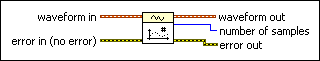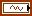waveform in is the waveform for which you want to retrieve the number of data elements.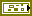error in describes error conditions that occur before this node runs. This input provides standard error in functionality.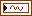waveform out returns waveform in unchanged.number of samples is the number of data elements in the waveform.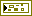error out contains error information. This output provides standard error out functionality.

## WDT Number of Waveform Samples CDBwaveform in is the waveform for which you want to retrieve the number of data elements.error in describes error conditions that occur before this node runs. This input provides standard error in functionality.waveform out returns waveform in unchanged.number of samples is the number of data elements in the waveform.error out contains error information. This output provides standard error out functionality.

## WDT Number of Waveform Samples EXTwaveform in is the waveform for which you want to retrieve the number of data elements.error in describes error conditions that occur before this node runs. This input provides standard error in functionality.waveform out returns waveform in unchanged.number of samples is the number of data elements in the waveform.error out contains error information. This output provides standard error out functionality.

## WDT Number of Waveform Samples I16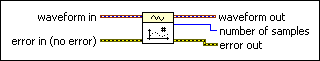waveform in is the waveform for which you want to retrieve the number of data elements.error in describes error conditions that occur before this node runs. This input provides standard error in functionality.waveform out returns waveform in unchanged.number of samples is the number of data elements in the waveform.error out contains error information. This output provides standard error out functionality.

## WDT Number of Waveform Samples I32waveform in is the waveform for which you want to retrieve the number of data elements.error in describes error conditions that occur before this node runs. This input provides standard error in functionality.waveform out returns waveform in unchanged.number of samples is the number of data elements in the waveform.error out contains error information. This output provides standard error out functionality.

## WDT Number of Waveform Samples I8waveform in is the waveform for which you want to retrieve the number of data elements.error in describes error conditions that occur before this node runs. This input provides standard error in functionality.waveform out returns waveform in unchanged.number of samples is the number of data elements in the waveform.error out contains error information. This output provides standard error out functionality.

## WDT Number of Waveform Samples SGLwaveform in is the waveform for which you want to retrieve the number of data elements.error in describes error conditions that occur before this node runs. This input provides standard error in functionality.waveform out returns waveform in unchanged.number of samples is the number of data elements in the waveform.error out contains error information. This output provides standard error out functionality.